Updated date:

# Using the Moving Average Tool from the Excel 2007 and Excel 2010 Analysis ToolPak

## Introduction

Welcome to my latest hub on the Analysis ToolPak in both Excel 2007 and Excel 2010. Today, I will look at how to use the Moving Average tool. This tool is used when you want to perform trend analysis on a sequence of data. In my example, I am looking at the number of overall hits my hubs get on a daily basis. I want to determine if my daily hits are trending upwards and the Moving Average Tool will create a trend line as part of the analysis to show me if this is the case (hopefully it is).

Moving averages use an interval to calculate the average over time. The interval chosen is the number of values Excel 2007 or Excel 2010 will average to create the trend line. I will go through how to determine the best interval to fit your data and give you the most accurate and meaningful trend line.

Before beginning, I have a hub that covers adding the Analysis ToolPak to Excel if it is not installed and also troubleshooting if it is installed but does not appear in Excel 2007 or Excel 2010. The hub can be found here:

http://robbiecwilson.hubpages.com/hub/Using-the-Analysis-ToolPak-in-Excel-2007-and-Excel-2010

When we have completed our analysis on the data using the Moving Average tool, we will be provided with a graph showing our actual data and the trend that the tool has calculated.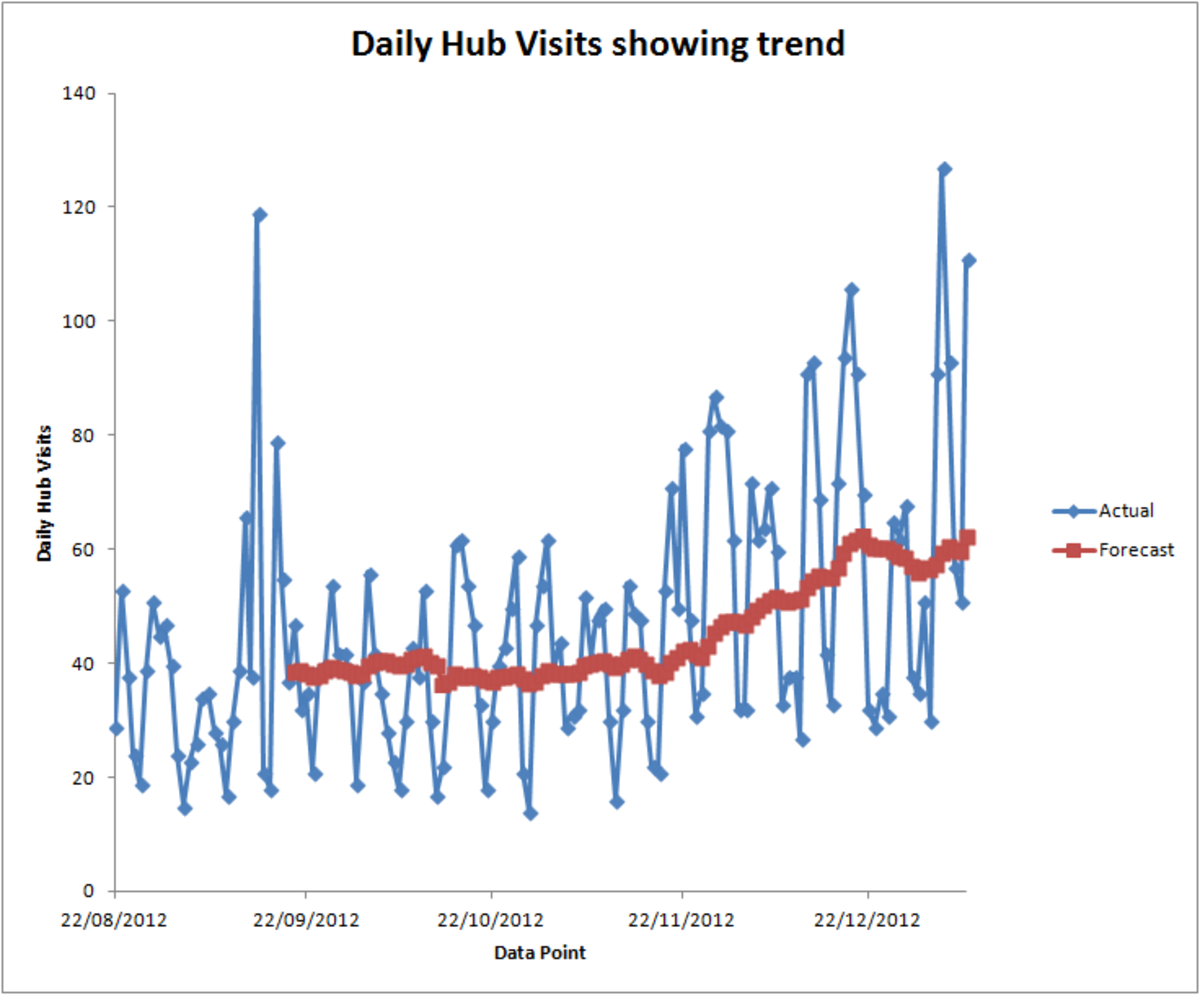Example of a Moving Average with a trend line, created using the Moving Average Tool from the Analysis Toolpak in Excel 2007 and Excel 2010.http://robbiecwilson.hubpages.com/

## Using the Moving Average Tool in Excel 2007 and Excel 2010

Provided you have the Analysis ToolPak installed in either Excel 2007 or Excel 2010, the ToolPak can be found on the Data tab, in the Analysis group.

To use it, simply click the Data Analysis button (you do not have to select any data before hand).

• Next, we select Moving Average from the list of available Analysis Tools
• The Moving Average dialogue box will now open
• To begin, select the Input Range. The range must be in a column and must also be contiguous (have no breaks). This also includes your labels should you select them
• Press Return or Enter to select the range
• Select the option for Labels in First Row if you have labels in that first row
• For Interval, leave this at the default of 3. We will discuss intervals in more detail in a further section below
• Select an Output Range under Output options (in my example, I chose the next column to keep it simple
• Select Chart Output so that Excel 2007 or Excel 2010 will create a chart for you as this is most likely what you will refer to most when using the Moving Average tool
• Leave Standard Errors blank (this creates a second column that contains the data) as it is unlikely that you will use it
• Click OK and Excel will create a column containing the moving averages and a chart

Note: The chart that is created is for some reason very small, you will need to re-size it to be able to actually see the detail. The figure below will give you an idea of how it looks once Excel has completed the analysis on your data.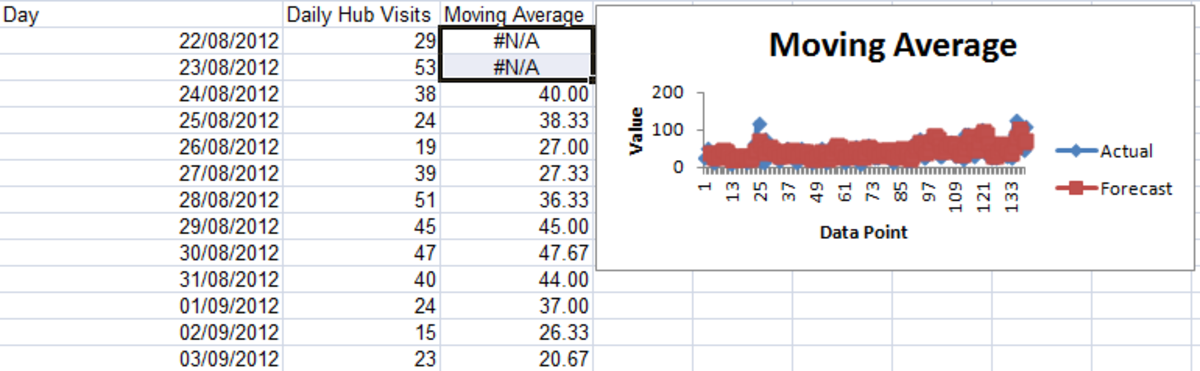Example of the initial output created using the Moving Average Tool from the Analysis Toolpak in Excel 2007 and Excel 2010.http://robbiecwilson.hubpages.com/

Note: As you can see from the figure above, the first two cells in the Moving Average column (I have highlighted the cells) have #N/A in them. This is normal as Excel cannot perform the moving average until it has three values (the interval in other words).

I have tidied up the chart below to show my data with a moving average of three days. I have a hub that covers creating and editing charts in much greater detail which can be found here:

http://robbiecwilson.hubpages.com/hub/Creating-charts-and-graphs-in-Excel-2007

As you can see, the trend line (forecast) is very variable and the trend is not that easy to see. We will discuss selecting an appropriate interval in next the section.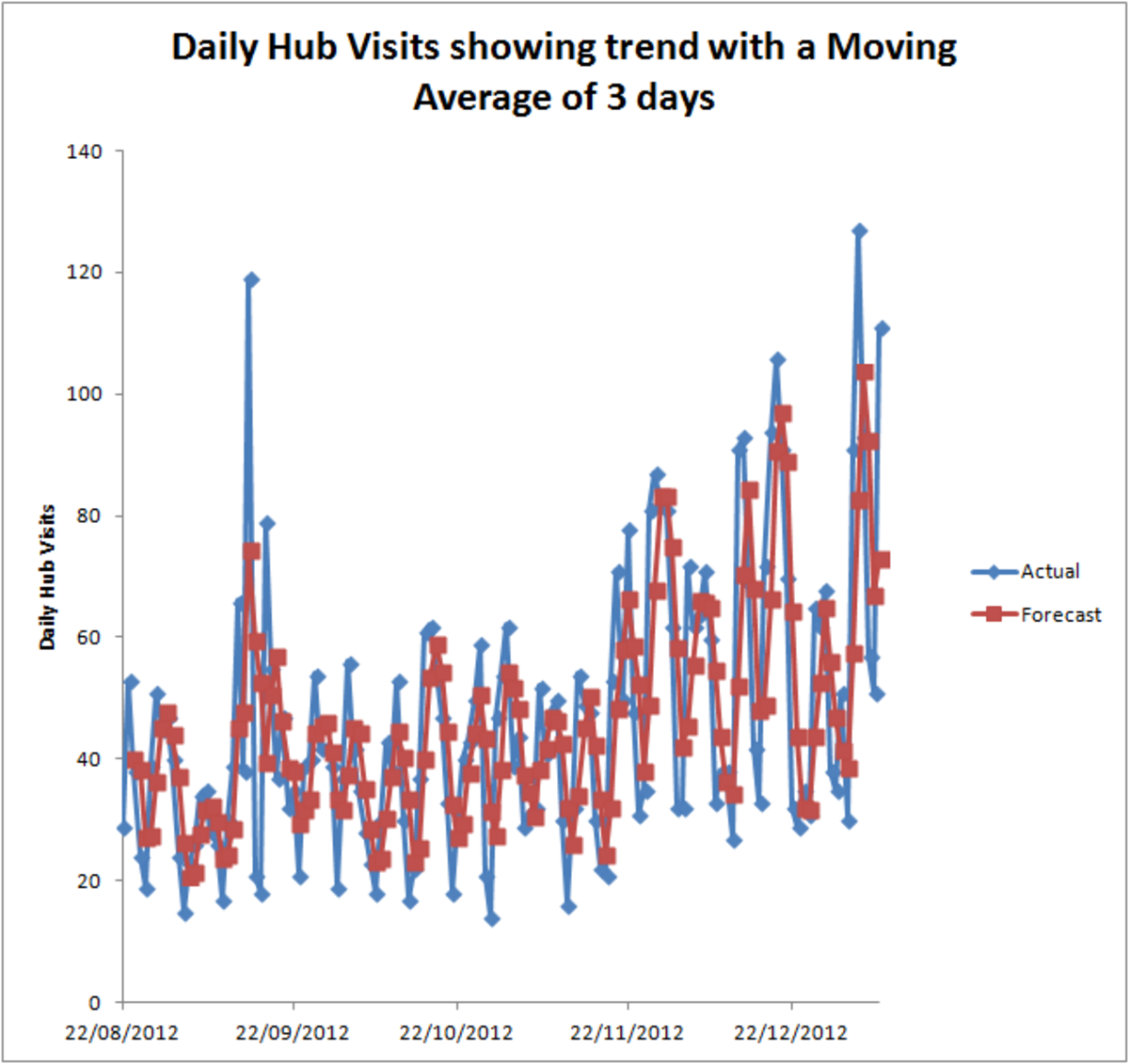Moving Average with an interval that does not show a definitive trend created using the Moving Average Tool from the Analysis Toolpak in Excel 2007 and Excel 2010.http://robbiecwilson.hubpages.com/

## Choosing the correct interval for your moving average in Excel 2007 and Excel 2010

As you can see from the figure above, the moving average is extremely variable and does not illustrate a useful trend or forecast. Getting the correct interval is crucial so that you can easily see the trend in your data.

Clearly in my example, using a forecast with an interval of three days would not provide me with any valuable data. The trend is becoming evident in the second chart in the figure below with the interval of seven day and is most clear in the thirty day moving average, so that is the one I would choose.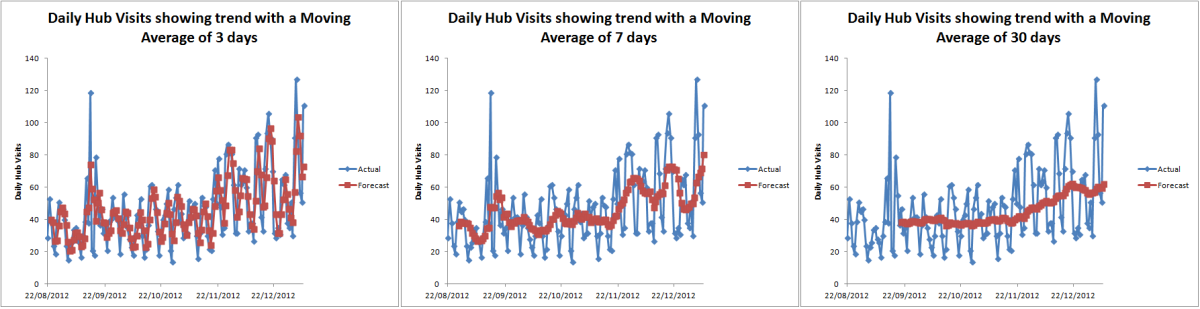Moving Averages with different intervals created using the Moving Average Tool from the Analysis Toolpak in Excel 2007 and Excel 2010.http://robbiecwilson.hubpages.com/

Two things become immediately apparent from those graphs.

1. The trend becomes clearer, the higher the interval
2. The data series needs to be longer if you choose a longer interval (the gap between the start of the data series and the start of the trend line becomes greater the higher interval you choose)

In order to choose the most appropriate interval you need to balance the two factors above, choosing a sufficiently long interval to show the best trend line based on the amount of data you have.

## Adding a trend line to a chart in Excel 2007 and Excel 2010

There is another method to add a trend line to a chart. This has advantages and disadvantages to using the Moving Average tool (I have added a trend line to my data in the figure below):

• Adding a trend line allows you to forecast backwards and forwards
• The line is linear and much tidier (useful to show the trend in a presentation)
• Very easy to add to an existing chart

• The underlying data used to create the trend line is not available
• Standard Error calculation is not available

So in summary, if you want a nice straight line showing trends either forwards or backwards, use a trend line. If you are interested in the underlying mathematics or the data of the moving averages, use the more powerful Moving Average tool from the Analysis ToolPak.

I also have a hub that investigates creating trend lines to charts in much greater detail, that hub can be found here:

## Conclusion

Moving averages is a useful and powerful mathematical tool for calculating a trend in a data series. Using the Moving Average tool from the Analysis ToolPak in Excel 2007 and Excel 2010, I was able to show that the daily traffic coming into my hubs is indeed trending upwards.

In this hub, I illustrated:

• How to use the Moving Average tool and also
• Looked at how to choose an appropriate interval for your data
• I also discussed adding a trend line to an existing chart and compared using this to using the Moving Average tool

I have a number of hubs covering other popular tools from the Analysis ToolPak in both Excel 2007 and Excel 2010. These include: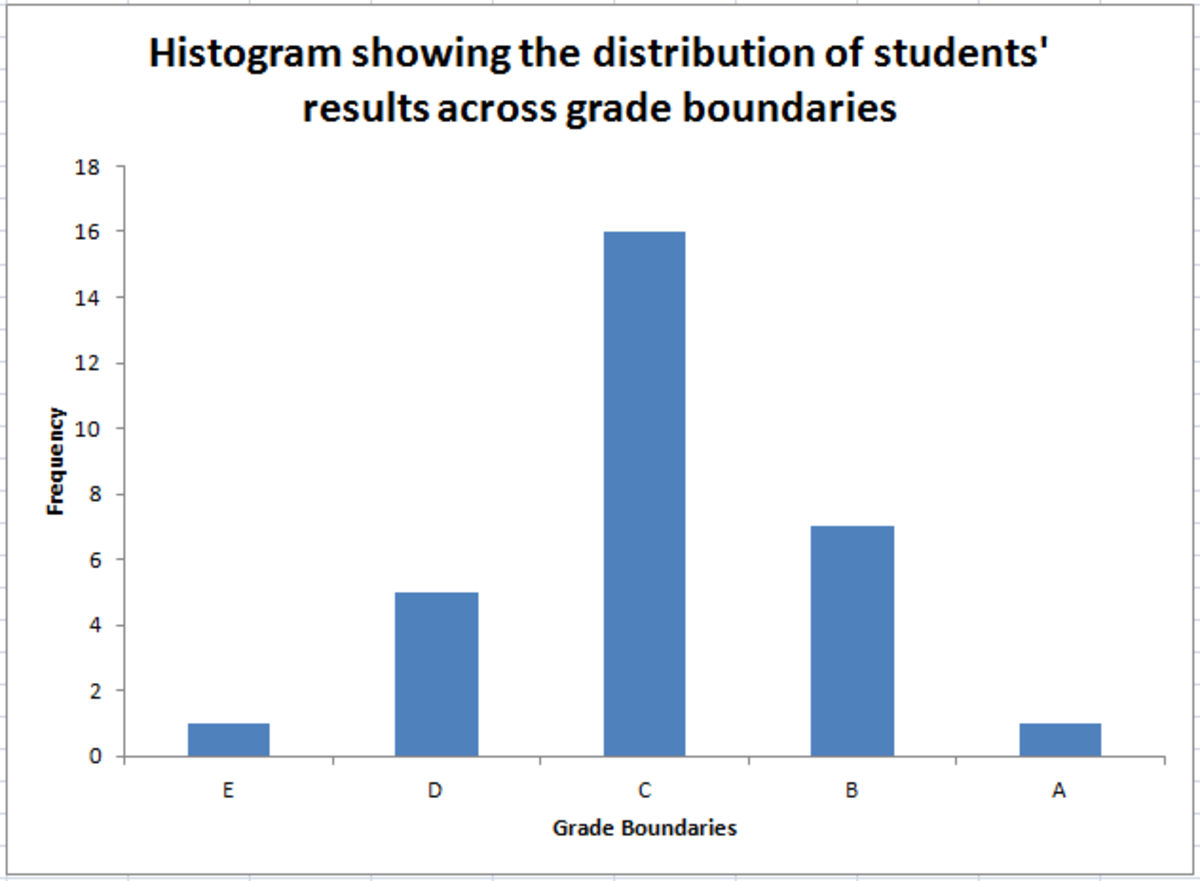Example of a Histogram, created using the Histogram Tool from the Analysis Toolpak in Excel 2007 and Excel 2010.http://robbiecwilson.hubpages.com/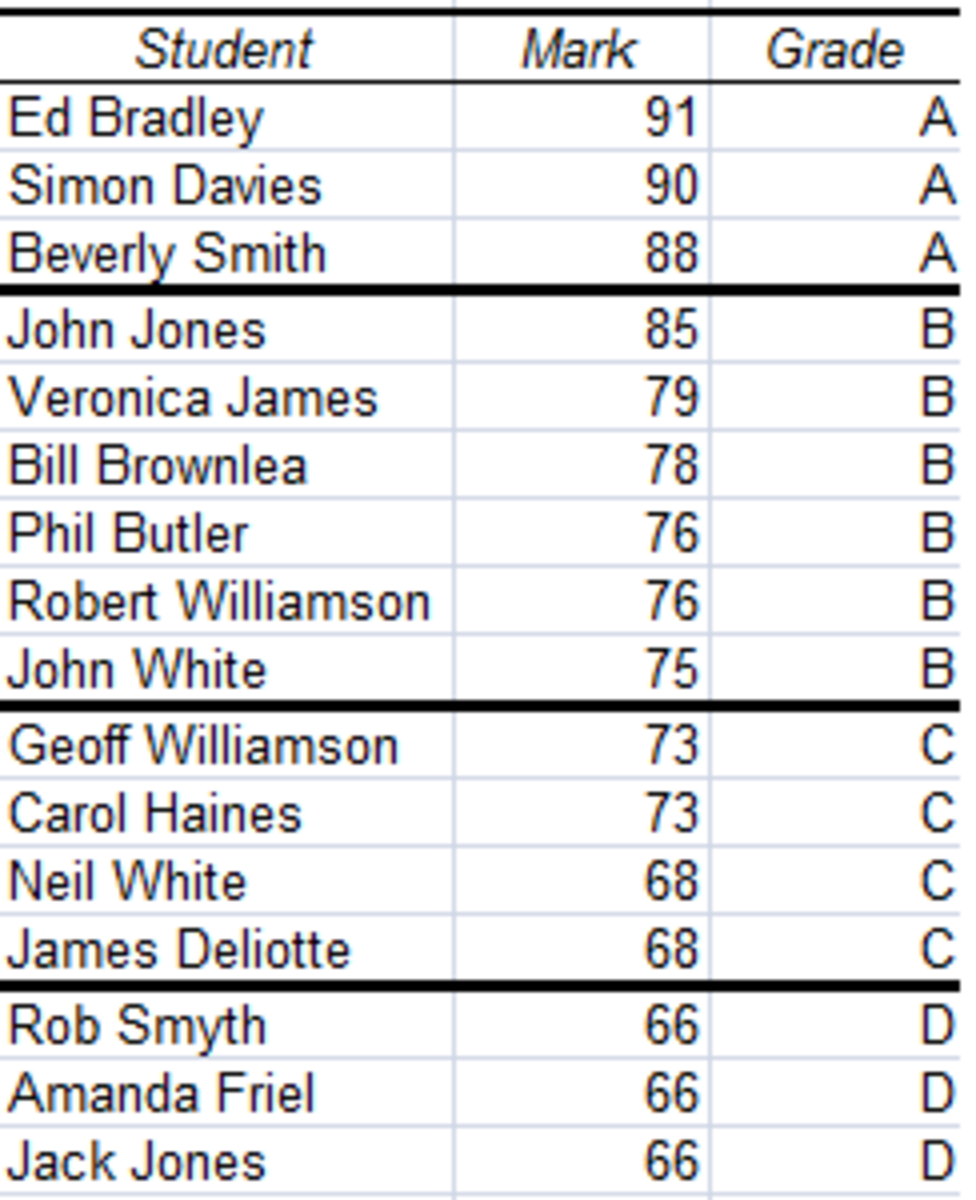Example of a Ranking Table, created using the Rank and Percentile Tool from the Analysis Toolpak in Excel 2007 and Excel 2010.http://robbiecwilson.hubpages.com/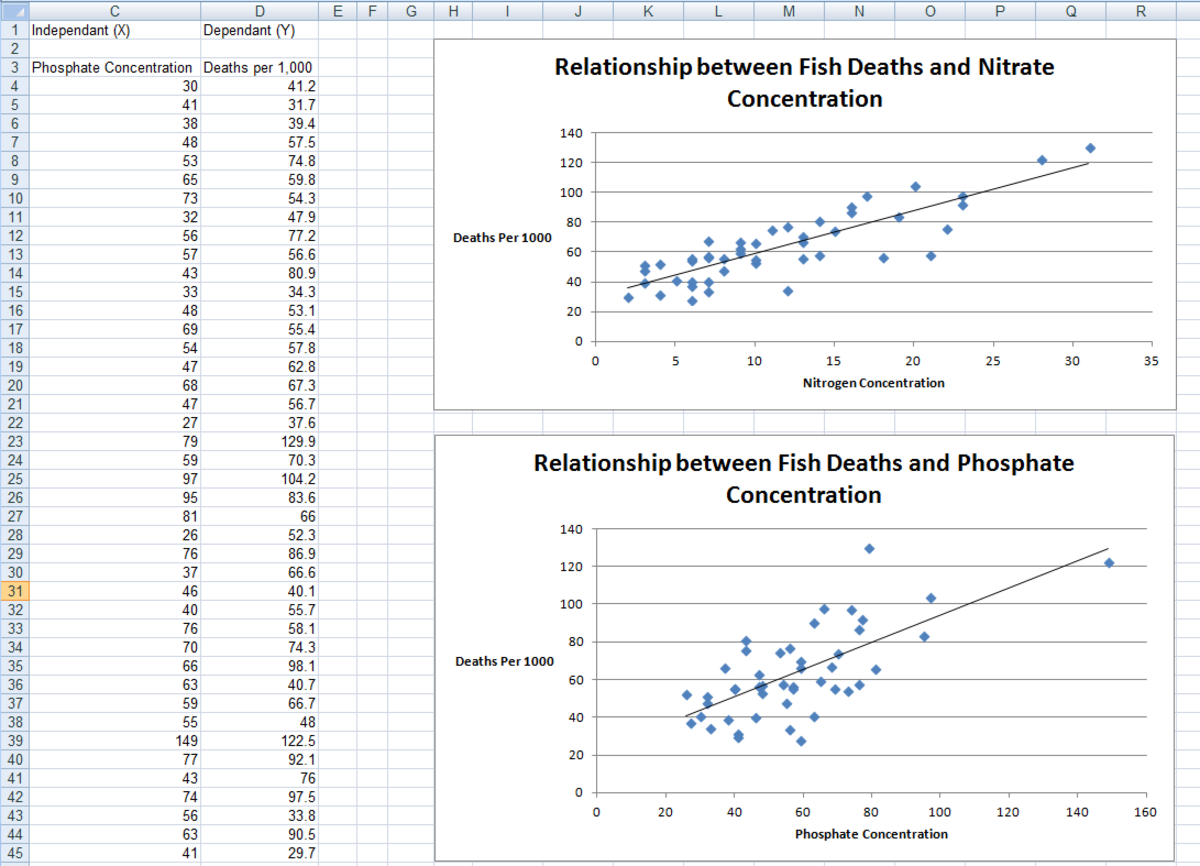Example of a Regression, created using the Regression Tool from the Analysis Toolpak in Excel 2007 and Excel 2010.http://robbiecwilson.hubpages.com/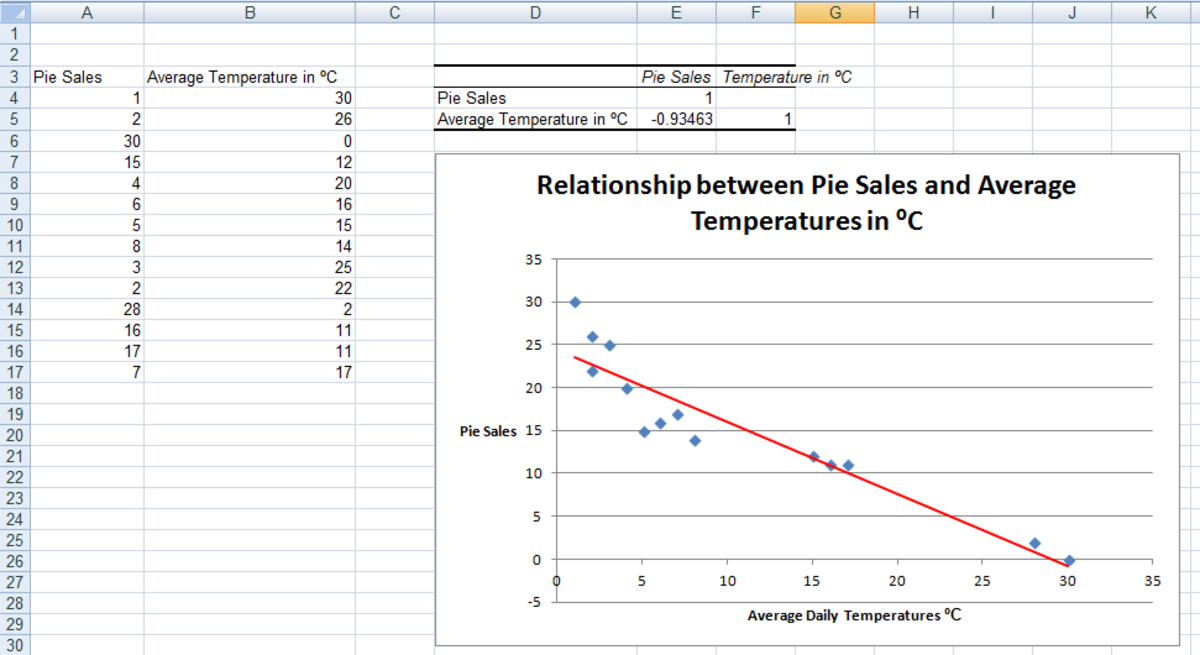Example of a Correlation, created using the Correlation Tool from the Analysis Toolpak in Excel 2007 and Excel 2010.http://robbiecwilson.hubpages.com/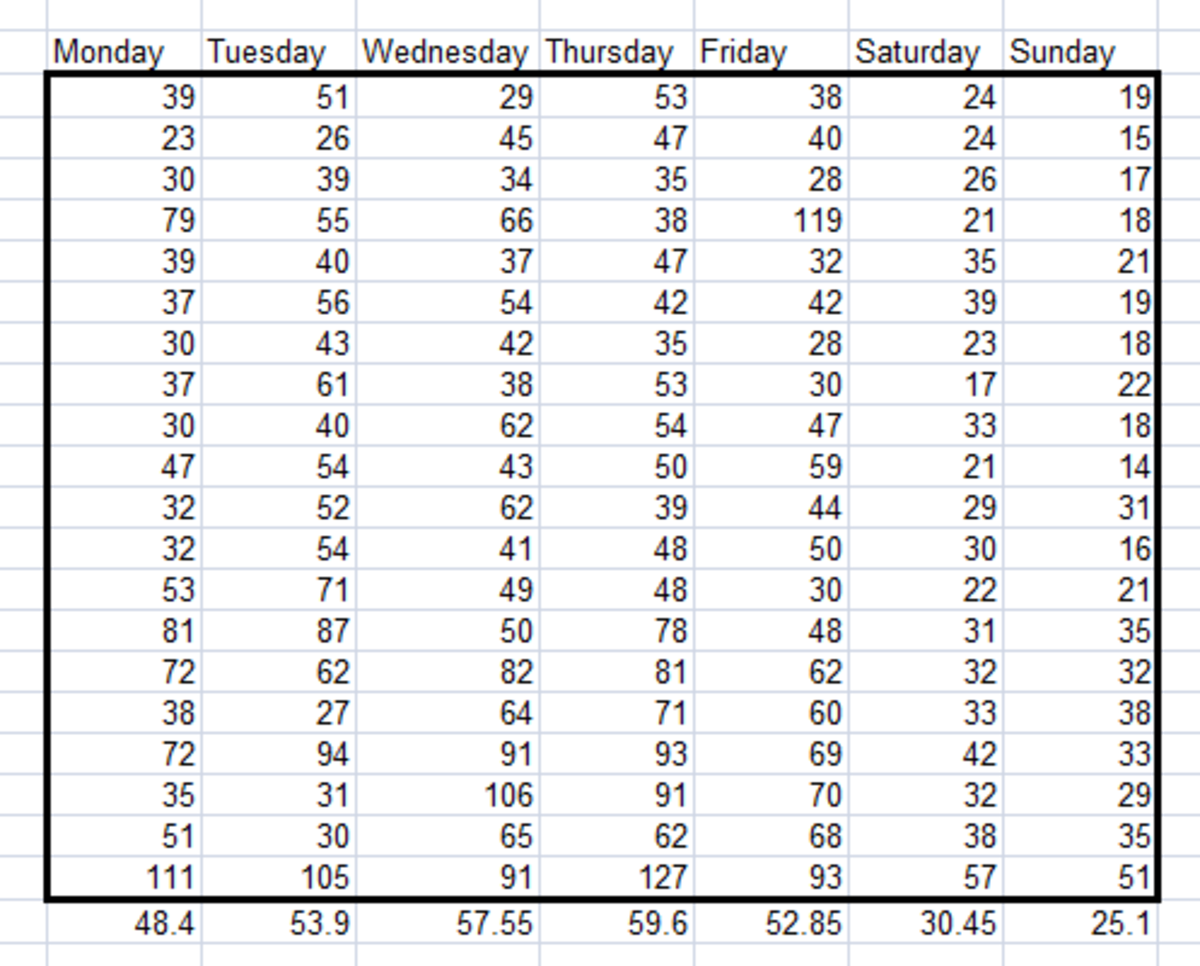Example of a table showing daily variation, created using the Sampling Tool from the Analysis Toolpak in Excel 2007 and Excel 2010.

Histogram: is another tool that creates a chart of your data. This tool looks at the distribution of your data across boundaries you define. In my hub on this tool, I look at the distribution of exam results across grade boundaries:

http://robbiecwilson.hubpages.com/hub/Using-the-Histogram-Tool-from-the-Analysis-ToolPak-in-Excel-2007-and-Excel-2010

Rank and Percentile is used to rank your data and assign a percentile to each unique value. I used this tool to rank students exam results and assign them a grade based on their position in that ranked list:

http://robbiecwilson.hubpages.com/hub/Use-the-Rank-and-Percentile-Tool-from-the-Analysis-ToolPak-in-Excel-2007-and-Excel-2010-to-create-ranking-tables

Correlation and Regression look at the relationship between variables. Correlation measures the strength of a relationship and regression creates a line that shows this relationship. In my hub on correlation, I examine the relationship between daily temperatures and pie sales and in my hub on regression, I look at the relationship between fish mortality and Phosphate and Nitrogen concentrations in water.

http://robbiecwilson.hubpages.com/hub/Using-the-Correlation-Tool-from-the-Excel-2007-and-Excel-2010-Analysis-ToolPak

http://robbiecwilson.hubpages.com/hub/Using-the-Regression-Tool-from-the-Analysis-ToolPak-in-Excel-2007-and-Excel-2010

Sampling allows you to create a randomly chosen sample from a population and perform analysis on it. I use sampling to pick lottery numbers in my hub.

http://robbiecwilson.hubpages.com/hub/Use-the-Sampling-Tool-in-the-Excel-2007-and-Excel-2010-Analysis-ToolPak-for-sampling-and-to-analyse-periodic-variations

Many thanks for reading and I hope that enjoyed reading this as much as I enjoyed writing it and that you found it useful and informative. Please feel free to leave any comments you may have below.